### Home > CALC > Chapter 6 > Lesson 6.5.1 > Problem6-168

6-168.
1. Determine what makes the integrals below "improper." Then, determine how to rewrite the integral using "proper" limit notation. Finally, find the area represented by each. Homework Help ✎

1.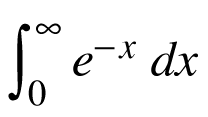2.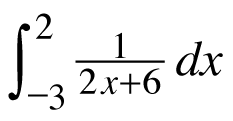3.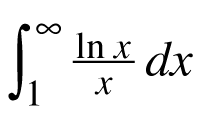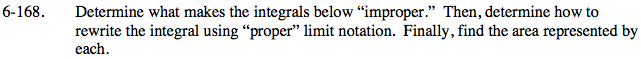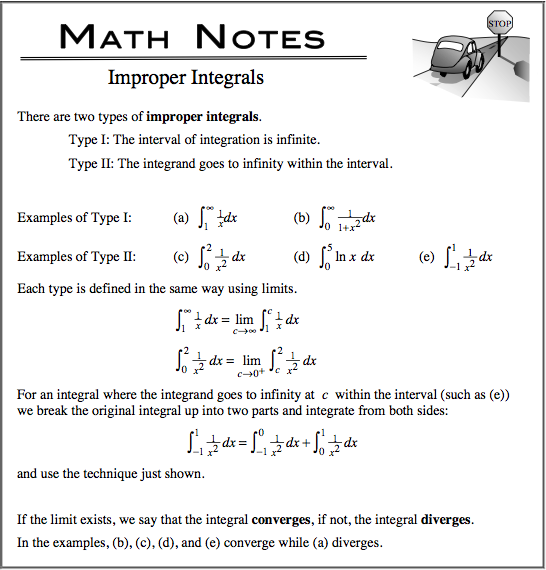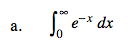Does the graph of the integrand, y = ex, have any asymptotes on the bounded domain, [0, ∞)?

$\lim_{a\to \infty }\int_{0}^{a}e^{-x}dx=\lim_{a\to \infty }-e^{-x}\Big|_0^a=\underline{\ \ \ \ \ \ \ }$

The answer should be very pleasing.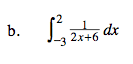$\text{Does the graph of the integrand, }y= \frac{1}{2x-6},$

have any asymptotes on the bounded domain, [–3, 2]?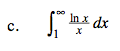Before you rewrite as a limit, use U-substitution on the integrand and the bounds. Let U = ln(x).